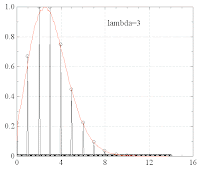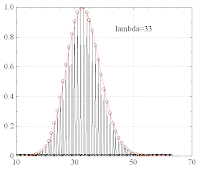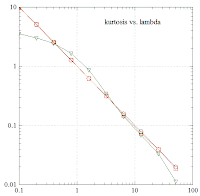## Wednesday, April 30, 2008

### [MT 6] Continuous Poisson distribution

It would be useful to have a generalization of the Poisson distribution for continuous event numbers k. This can be achieved by replacing the factorial by the gamma-function:

The following plot compares the discrete PDF (black) with the continuous one (orange) for lambda=3:The same for lambda=33:Theoretically, the kurtosis of the discrete Poisson distribution is 1/lambda. The following plot compares the numerical kurtosis=f(lambda) in the discrete (orange) and in the continuous version (green) with the analytical expectation (black):The continuous Poisson distribution is obviously related to the gamma distribution with a=k+1. However, in the gamma distribution with shape parameter a and rate parameter b,

it is x=lambda which is considered as the random variable.

#### 5 comments:

1.Hi, what about normalization? I could not find any analytic result that helped me integrate \lambda^k / \Gamma(k+1) .

2.Great! Thanks

3.Hi, do you know how to sample from the continuous Poisson distribution, i.e. k is replaced by a continuous variable x?

It seems MATLAB or R can only generate random numbers from discrete Poisson distribution. Where is a generator for the continuous version?

4.Hi, do you know how to sample from the continuous Poisson distribution, i.e. k is replaced by a continuous variable x?

It seems MATLAB or R can only generate random numbers from discrete Poisson distribution. Where is a generator for the continuous version?

1.There is no continuous Poisson distribution. What has been discussed is a potential continuous extension of the Poisson. So it is not surprising that MATLAB or R will have only the discrete distribution. However, you may write your own little code to draw samples from a uniform distribution, then apply the inverse function to get random numbers from any distribution you please.